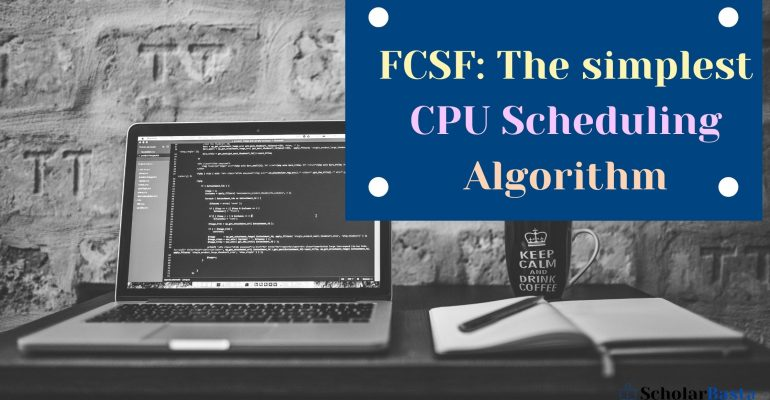## FCSF: The simplest CPU Scheduling Algorithm# FCSF: The simplest CPU Scheduling Algorithm

### What is CPU Scheduling?

Scheduling (CPU scheduling) is the process of assigning different jobs or processes to the CPU to make the system more efficient, productive, fast, and fair.

The process of CPU scheduling forms the basis of Multiprogrammed operating systems.

CPU scheduling is done by the Operating System with the help of short-term scheduler (CPU scheduler).

This CPU scheduler selects a process among the processes maintained in the ready queue (main memory) and assigns it to the CPU based on the chosen set of criteria for its execution.

### Some Important terms related to CPU scheduling

• Arrival Time: It is the point of time at which the process arrives in the main memory (ready queue).
• Burst/Execution Time: It is the time duration for which the process gets executed by the CPU and remains in the running state.
• Completion Time: It is the point of time at which the process completes its execution.
• Turn Around Time: It is the time duration for which the process stays in the main memory.
• (Turn Around Time = Completion Time – Arrival Time)
• Waiting Time: It is the time duration for which the process has to wait before it gets assigned to the CPU.
• (Waiting Time = Turn Around Time – Burst Time )
• Response Time: It is the difference between the time at which the process gets the CPU for the first time and the arrival time of the process.
• (Response Time = The time at which the process first gets the CPU – Arrival Time)
• Average Turn Around Time: (Sum of Turn Around Time of all processes) / (Number of processes)
• Average Waiting Time: (Sum of Waiting Time of all processes) / (Number of processes)
• Average Response Time: (Sum of Response Time of all processes) / (Number of processes)

### Types of CPU Scheduling

There are mainly six types of scheduling algorithms which improves the system performance based on certain criteria.

1. First Come First Serve (FCFS)
2. Shortest Job First (SJF)
3. Shortest Remaining Time First (SRTF)
4. Priority Scheduling
5. Round Robin Scheduling
6. Multilevel Queue Scheduling
##### But here in this tutorial, we will discuss the simplest CPU scheduling Algorithm that is First Come First Serve (FCFS).

Also Read Introduction to C++ STL

### What is FCFS?

This is the simplest CPU scheduling algorithm used for scheduling the processes maintained in the ready queue or main memory.

In this scheduling algorithm, the processes in the ready queue are scheduled based on their **arrival time.

FCFS is a **Non-pre-emptive scheduling algorithm.

Non-preemptive scheduling algorithm: In the case of the non-preemptive scheduling algorithm the execution of the next process starts only when the currently executing process completes its execution and gets terminated. No context switching takes place in the case of the non-preemptive scheduling algorithm. No process is interrupted during its execution.

NOTE: In case of all the non-preemptive scheduling algorithms, Waiting Time = Response Time.

### C++ program to implement the FCFS scheduling algorithm

``````#include<iostream>

using namespace std;

int main()
{
int N;
cout<<"Enter the No. of processes: ";
cin>>N;
/*pid -> process id
at -> arrival time
bt -> burst time
ct -> completion time
tat -> turnaround time
wt -> waiting time
rt -> response time*/

int at[N],bt[N],ct[N],tat[N],wt[N],rt[N];
float avg_wt = 0,avg_tat = 0, avg_rt = 0;

// Input Burst Time and Arrival Time
for(int i=0; i<N; i++)
{
cout<<"\nEnter Arrival Time & Burst Time for P"<<i+1<<": ";
cin>>at[i]>>bt[i];
cout<<endl;
}

// Completion Time
ct = bt;
for(int i=1; i<N; i++)
{
ct[i] = ct[i-1] + bt[i];
}

// Turnaround Time
for(int i=0; i<N; i++)
{
tat[i] = ct[i] - at[i];
avg_tat = avg_tat + tat[i];
}
avg_tat = avg_tat / N;

// Waiting Time
for(int i=0; i<N; i++)
{
wt[i] = tat[i] - bt[i];
avg_wt = avg_wt + wt[i];
}
avg_wt = avg_wt / N;

// Response Time
for(int i=0; i<N; i++)
{
rt[i] = wt[i];
avg_rt = avg_rt + rt[i];
}
avg_rt = avg_rt / N;

// Print Table of PID, AT, CT, BT, TAT, WT, & RT
cout<<"\nPID\tAT\tBT\tCT\tTAT\tWT\tRT"<<endl;
for(int i=0; i<N; i++)
{
cout<<endl;
cout<<"P"<<i+1<<"\t"<<at[i]<<"\t"<<bt[i]<<"\t"<<ct[i]<<"\t"<<tat[i
]<<"\t"<<wt[i]<<"\t"<<rt[i];
}
cout<<endl;
// Average TAT, WT & RT
cout<<"\nAverage Turnaround Time: "<<avg_tat;
cout<<"\nAverage Waiting Time: "<<avg_wt;
cout<<"\nAverage Response Time: "<<avg_rt;
cout<<endl;
return 0;
}``````

Output:

`````` Enter the No. of processes: 3
Enter Arrival Time & Burst Time for P1: 0 9
Enter Arrival Time & Burst Time for P2: 1 5
Enter Arrival Time & Burst Time for P3: 2 7
PID     AT      BT      CT      TAT     WT      RT
P1      0       9       9       9       0       0
P2      1       5       14      13      8       8
P3      2       7       21      19      12      12
Average Turnaround Time: 13.6667
Average Waiting Time: 6.66667
Average Response Time: 6.66667``````